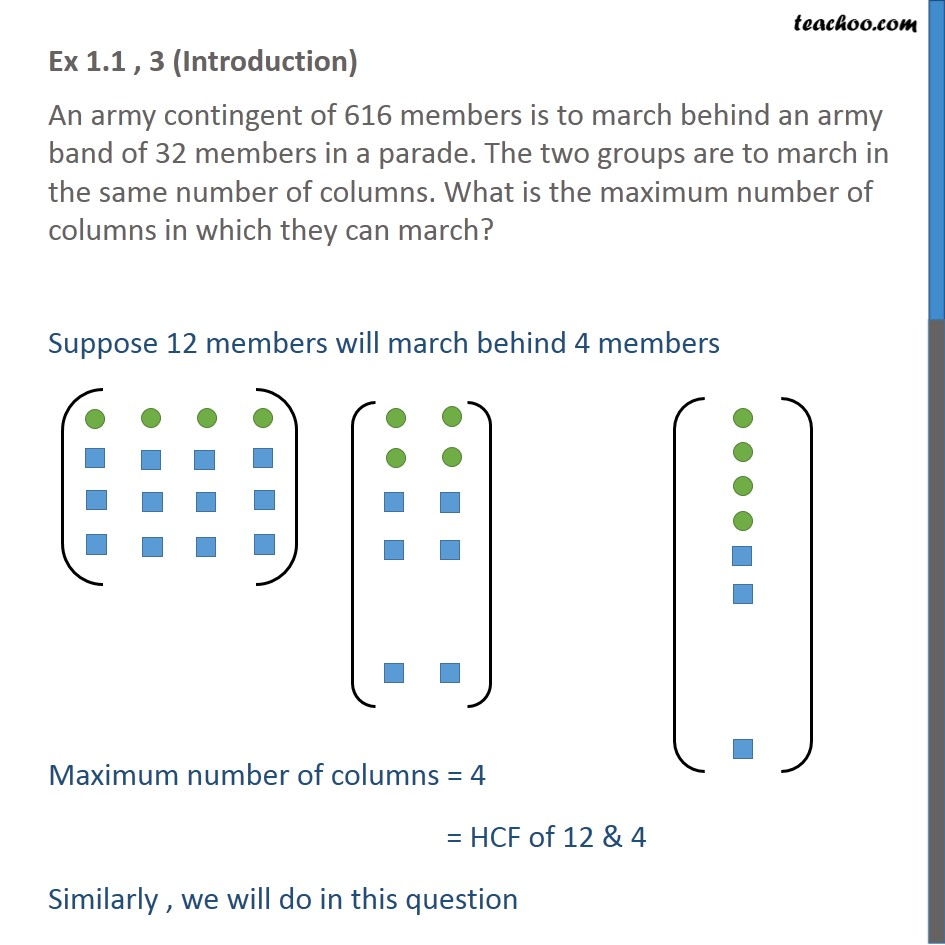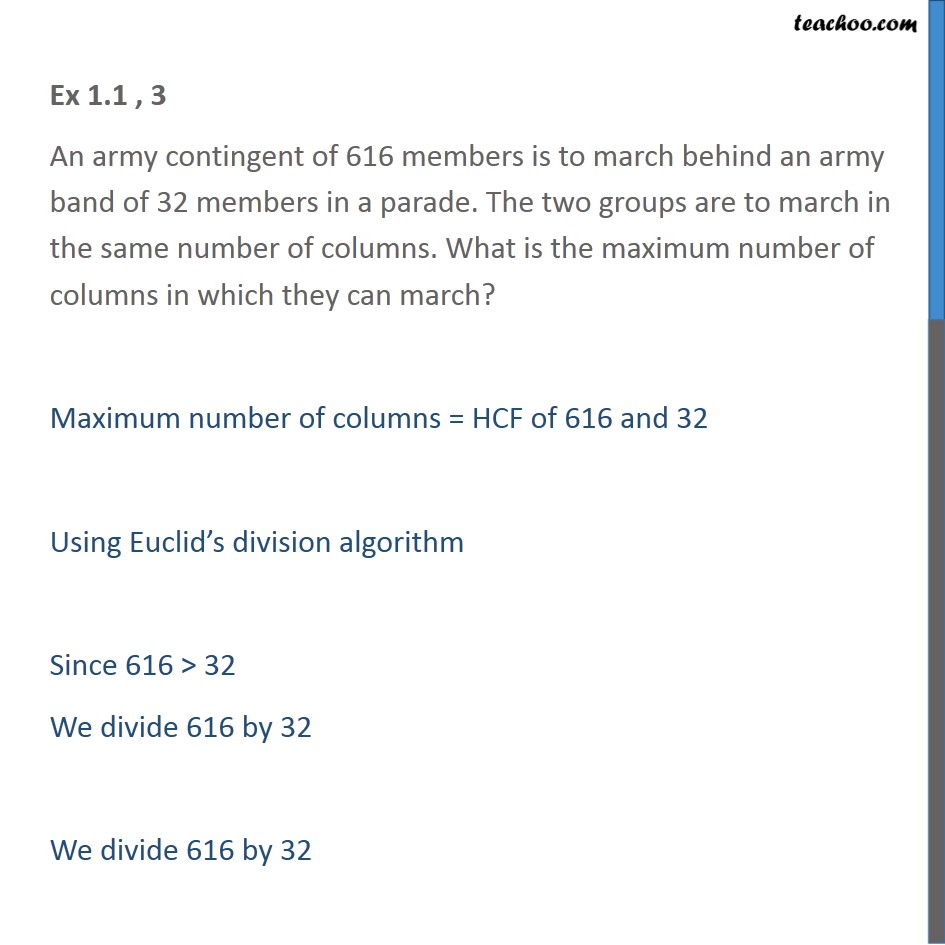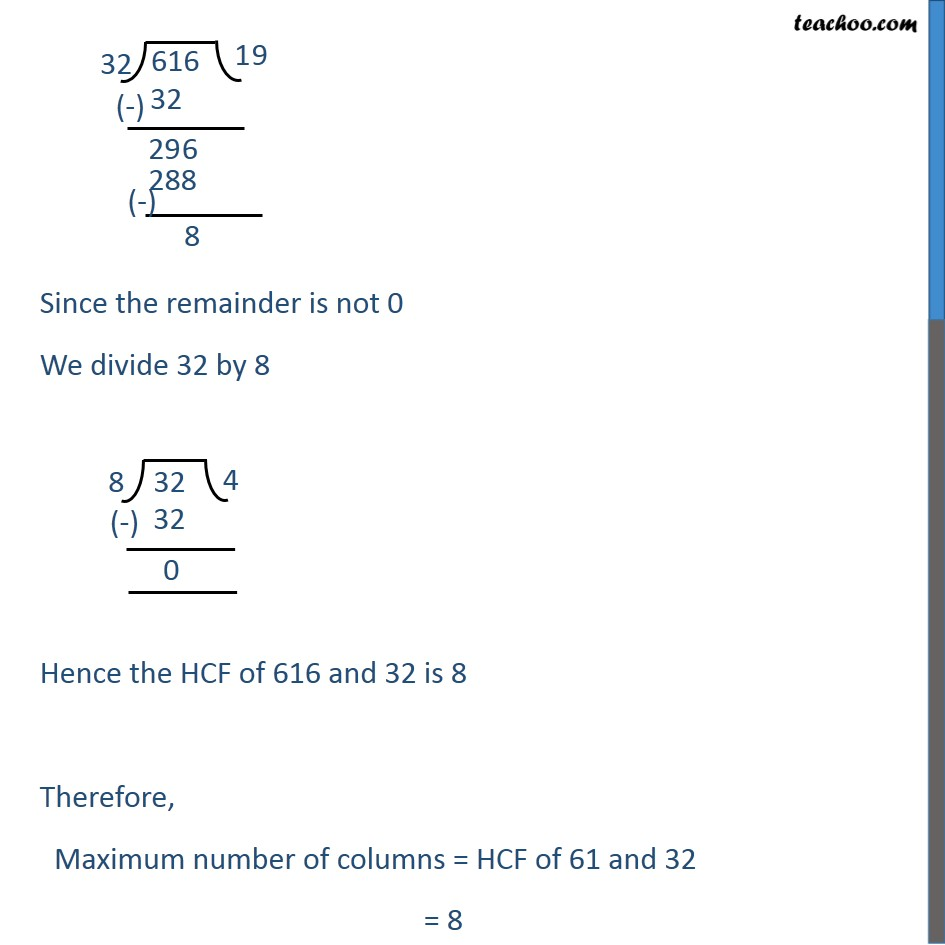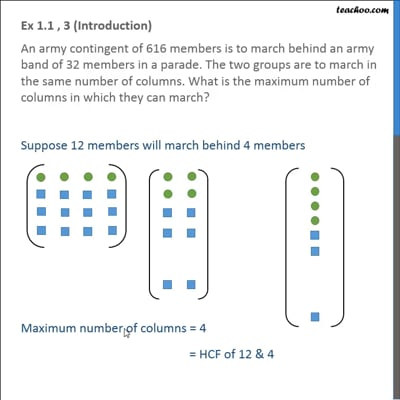Ex 1.1

Chapter 1 Class 10 Real Numbers
Serial order wiseThis video is only available for Teachoo black users

Solve all your doubts with Teachoo Black (new monthly pack available now!)

### Transcript

Ex 1.1 , 3 (Introduction) An army contingent of 616 members is to march behind an army band of 32 members in a parade. The two groups are to march in the same number of columns. What is the maximum number of columns in which they can march? Suppose 12 members will march behind 4 members Maximum number of columns = 4 = HCF of 12 & 4 Similarly , we will do in this question Ex 1.1 , 3 An army contingent of 616 members is to march behind an army band of 32 members in a parade. The two groups are to march in the same number of columns. What is the maximum number of columns in which they can march? Maximum number of columns = HCF of 616 and 32 Using Euclid’s division algorithm Since 616 > 32 We divide 616 by 32 We divide 616 by 32 Since the remainder is not 0 We divide 32 by 8 Hence the HCF of 616 and 32 is 8 Therefore, Maximum number of columns = HCF of 61 and 32 = 8